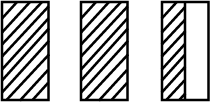Courses
Courses for Kids
Free study material
Offline Centres
MoreLast updated date: 08th Dec 2023
Total views: 281.1k
Views today: 6.81k

# The following diagram represents how many shaded parts?(a) $\dfrac{3}{2}$(b) $2\dfrac{1}{3}$(C) $2\dfrac{1}{2}$(d) $3\dfrac{1}{2}$Verified
281.1k+ views
Hint: In this problem we are going to count the number of shaded rectangles from the given diagram. From the whole rectangle we are going to find only the shaded part. Thus, this question is based on part-whole. Part-whole is a ratio or a fraction that represents a relationship between a part and its whole.

Complete step-by-step solution:From the above diagram, we can get
The total number of rectangle is $3$
Count the number of fully shaded rectangle is $2$
Out of $3$, $2$ rectangles are fully shaded and only the half of the last rectangle is shaded. In mathematics full is represented by the number $1$, and the half is represented in fraction as $\dfrac{1}{2}$.
So, the total shaded area $= 2 + \dfrac{1}{2}$
Here we want to add two numbers, where the first number is a natural number and the other is fraction. To add both the numbers let convert the natural number into fraction. When there is no denominator for a number we can say that the denominator is $1$, because any number divided by $1$ is the number itself.
The total shaded area $= \dfrac{2}{1} + \dfrac{1}{2} = \dfrac{{2(2) + 1}}{2}$
$= \dfrac{{4 + 1}}{2}$
Total shaded area $= \dfrac{5}{2}$
Here, the fraction is in improper fraction so let convert it into mixed fraction, we will get,
Therefore, the total shaded area is $2\dfrac{1}{2}$
Hence, option (c) is the correct answer.

Note: A fraction is a part of a whole. When the question is in a mixed fraction we should change it into an improper fraction and then only start to simplify. If the answer we get is in an improper fraction, finally we should change it into a mixed fraction.